#### You may also like### Cubes

How many faces can you see when you arrange these three cubes in different ways?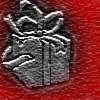### Wrapping Presents

Choose a box and work out the smallest rectangle of paper needed to wrap it so that it is completely covered.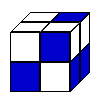### Cubic Conundrum

Which of the following cubes can be made from these nets?

# The Big Cheese

##### Age 7 to 11Challenge Level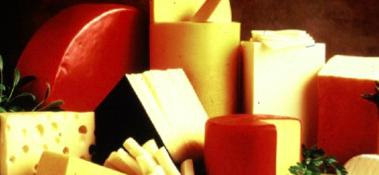I met up with some friends yesterday for lunch. On the table was a good big block of cheese. It looked rather like a cube. As the meal went on we started cutting off slices, but these got smaller and smaller! It got me thinking ...

What if the cheese cube was $5$ by $5$ by $5$ and each slice was always $1$ thick?

It wouldn't be fair on everyone else's lunch if I cut up the real cheese so I made a model out of multilink cubes: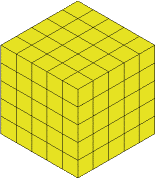##### You could of course, just make $5$ slices but I wanted to have a go at something else - keeping what is left as close to being a cube as possible.

You can see that it's a $5$ by $5$ by $5$ because of the individual cubes, so the slices will have to be $1$ cube thick.

So let's take a slice off the right hand side, I've coloured it in so you can see which bit I'm talking about:This now gets cut off and we have: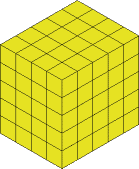The next slice will be from the left hand side (shown in a different colour again):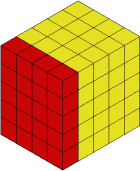Well the knife cuts and we are left with: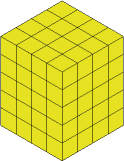#### Remember I'm setting myself the task of cutting so that I am left with a shape as close to a cube shape as possible each time.

So the next cut is from the top. Hard to cut this so I would have put it on its side!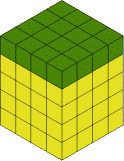I'll remove that and I'm left with the $4$ by $4$ by $4$ cube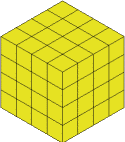I do three more cuts to get to the $3$ by $3$ by $3$ and these leave the block like this: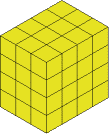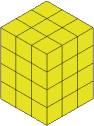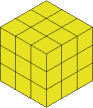I'm sure you've got the idea now so I don't need to talk as much about what I did: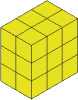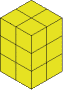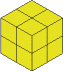and then onto: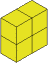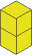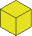That leaves you with two of the smallest size cube $1$ by $1$ by $1$.

If we keep all the slices and the last little cube, we will have pieces that look like (seen from above):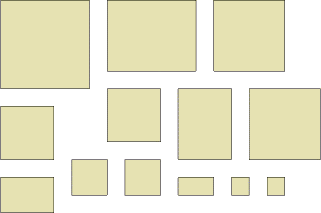#### C H A L L E N G E

Now we have thirteen objects to explore.
• What about the areas of these as seen from above?
• What about the total surface areas of these?
• What about their volumes of the pieces?

#### A L S O

Investigate sharing these thirteen pieces out so that everyone gets an equal share.

I guess that once you've explored the pattern of numbers you'll be able to extend it as if you had started with a $10$ by $10$ by $10$ cube of cheese.